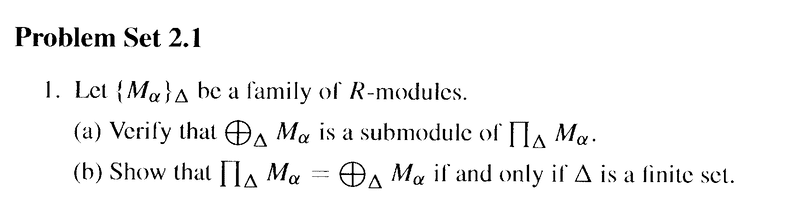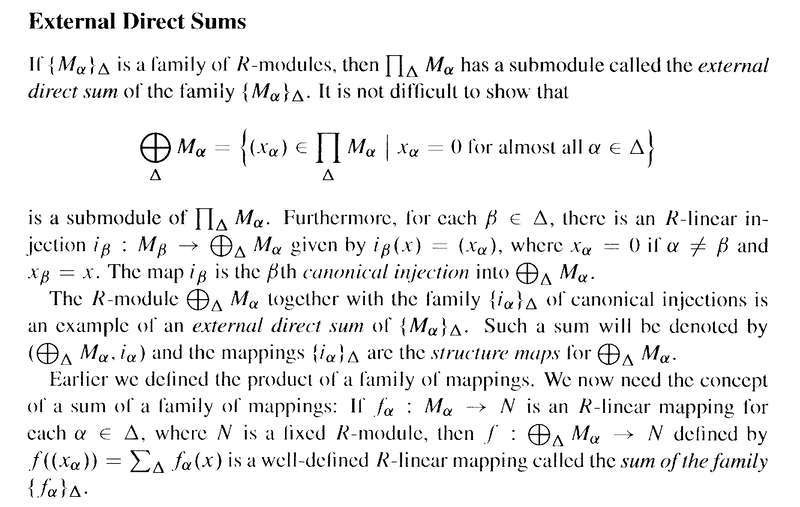# External Direct Sums & Direct Products .... Bland Ex. 1b, 2.1

Gold Member
MHB

## Homework Statement

I am reading Paul E. Bland's book: Rings and Their Modules and am currently focused on Section 2.1 Direct Products and Direct Sums ... ...

I need help with Problem 1(b) of Problem Set 2.1 ...

Problem 1(b) of Problem Set 2.1 reads as follows:

Bland Problem 1, Section 2.1 reads as follows:I need some help with 1(b) ...

## Homework Equations

The definition of External Direct Sum is as follows:## The Attempt at a Solution

I have had difficulty in formulating a rigorous and convincing proof of the statement in Problem 1(b) ... can someone please

(1) critique my attempt at a proof (see below)

(2) provide an alternate rigorous and convincing proof

My attempt at a proof is as follows:

We need to demonstrate that ##\prod_\Delta M_\alpha = \bigoplus_\Delta M_\alpha## if and only if ##\Delta## is a finite set ...

Assume ##\prod_\Delta M_\alpha = \bigoplus_\Delta M_\alpha##

The above equality would require all of the terms ##(x_\alpha)## of ##\prod_\Delta M_\alpha## to have a finite number of components or elements in each ##(x_\alpha)## ... thus ##\Delta## is a finite set ...

Assume ##\Delta## is a finite set

... then ##\prod_\Delta M_\alpha## has terms of the form ##(x_\alpha) = ( x_1, x_2, \ ... \ ... \ , x_n )## for some ##n \in \mathbb{Z}## ... ...

and

... ## \bigoplus_\Delta M_\alpha## has the same terms given that each of the above terms ##(x_\alpha)## has a finite number of components ...

Hope someone can indicate how to formulate a better proof ...

Peter

#### Attachments

Homework Helper
Gold Member
Let ##y\in \prod_{\alpha\in\Delta} M_\alpha##. Then ##y## is a function with domain ##\Delta## and range ##\bigcup_{\alpha\in\Delta} M_\alpha## such that ##y(\alpha)\in M_\alpha##. Define ##N(y)=\{\alpha\in\Delta\ :\ y(\alpha)\neq 0_{M_\alpha}\}##, which is the set of 'nonzero' components of ##y##. Then by definition ##y## is also in the direct sum ##\sum_{\alpha\in\Delta} M_\alpha## iff ##|N(y)|<\infty##, that is, if ##y## has only a finite number of nonzero components.

If ##\Delta## is finite then every ##y## has a finite number of components, hence a finite number of nonzero components, hence is in the direct sum.

To prove the reverse direction we want to show that if ##\Delta## is infinite, there exists a ##y## in the direct product that is not in the direct sum, ie which has an infinite number of nonzero components. In fact that will only be true if an infinite number of modules in ##\Delta## are nontrivial. If that is the case then, to construct our counterexample element ##y##, for every nontrivial module ##M_\alpha##, we choose a nonzero element in the ##\alpha## position. Then ##y## will have an infinite number of nonzero components and hence is not in the direct sum.

Note that that para uses the axiom of choice, which is always a little sad. We can avoid it if we are given some more info about each module, such as a basis. Say that we are given a generating-set function ##B:\Delta\times\mathbb N\to \bigcup_{\alpha\in\Delta} M_\alpha## such that ##B(\alpha,k)## is the ##k##th element of the specified generating-set for ##M_\alpha##, and ##0_{M_\alpha}## if ##k## is larger than the size of the generating set of ##\alpha##. Then we can construct our counterexample ##y## as ##y(\alpha)=B(\alpha,1)## without using the axiom of choice.

So, to state the theorem part (b) properly, we would say that the direct product of a set ##\Delta## of nontrivial modules is equal to the direct sum thereof if the set is finite and that, assuming the axiom of choice, if the direct product is equal to the direct sum, the set ##\Delta## must be finite.

It is possible we can avoid using the axiom of choice, but it is late and my brain is tired and I can't see how right now.

•Math Amateur
Gold Member
MHB
Let ##y\in \prod_{\alpha\in\Delta} M_\alpha##. Then ##y## is a function with domain ##\Delta## and range ##\bigcup_{\alpha\in\Delta} M_\alpha## such that ##y(\alpha)\in M_\alpha##. Define ##N(y)=\{\alpha\in\Delta\ :\ y(\alpha)\neq 0_{M_\alpha}\}##, which is the set of 'nonzero' components of ##y##. Then by definition ##y## is also in the direct sum ##\sum_{\alpha\in\Delta} M_\alpha## iff ##|N(y)|<\infty##, that is, if ##y## has only a finite number of nonzero components.

If ##\Delta## is finite then every ##y## has a finite number of components, hence a finite number of nonzero components, hence is in the direct sum.

To prove the reverse direction we want to show that if ##\Delta## is infinite, there exists a ##y## in the direct product that is not in the direct sum, ie which has an infinite number of nonzero components. In fact that will only be true if an infinite number of modules in ##\Delta## are nontrivial. If that is the case then, to construct our counterexample element ##y##, for every nontrivial module ##M_\alpha##, we choose a nonzero element in the ##\alpha## position. Then ##y## will have an infinite number of nonzero components and hence is not in the direct sum.

Note that that para uses the axiom of choice, which is always a little sad. We can avoid it if we are given some more info about each module, such as a basis. Say that we are given a generating-set function ##B:\Delta\times\mathbb N\to \bigcup_{\alpha\in\Delta} M_\alpha## such that ##B(\alpha,k)## is the ##k##th element of the specified generating-set for ##M_\alpha##, and ##0_{M_\alpha}## if ##k## is larger than the size of the generating set of ##\alpha##. Then we can construct our counterexample ##y## as ##y(\alpha)=B(\alpha,1)## without using the axiom of choice.

So, to state the theorem part (b) properly, we would say that the direct product of a set ##\Delta## of nontrivial modules is equal to the direct sum thereof if the set is finite and that, assuming the axiom of choice, if the direct product is equal to the direct sum, the set ##\Delta## must be finite.

It is possible we can avoid using the axiom of choice, but it is late and my brain is tired and I can't see how right now.

Hi Andrew ... thanks so much for the help ...

BUT ... I need further help to understand what you have said ...

You write:

" ... ... Let ##y\in \prod_{\alpha\in\Delta} M_\alpha##. Then ##y## is a function with domain ##\Delta## and range ##\bigcup_{\alpha\in\Delta} M_\alpha## such that ##y(\alpha)\in M_\alpha##. ... ... "

Can you please explain (slowly ...... ) how/why ##y## is a function with domain ##\Delta## and range ##\bigcup_{\alpha\in\Delta} M_\alpha## ...

Sorry to be slow to catch on ...

Peter

Mentor
2022 Award
Hi Andrew ... thanks so much for the help ...

BUT ... I need further help to understand what you have said ...

You write:

" ... ... Let ##y\in \prod_{\alpha\in\Delta} M_\alpha##. Then ##y## is a function with domain ##\Delta## and range ##\bigcup_{\alpha\in\Delta} M_\alpha## such that ##y(\alpha)\in M_\alpha##. ... ... "

Can you please explain (slowly ...... ) how/why ##y## is a function with domain ##\Delta## and range ##\bigcup_{\alpha\in\Delta} M_\alpha## ...

Sorry to be slow to catch on ...

Peter
It is an equivalent way of viewing direct products. In the book, the elements are ##(y_\alpha)_{\alpha \in \Delta}##, but what does that mean? It means we have for each ##\alpha## an element ##y_\alpha \in M_\alpha##. But this is as well a function, because it maps ##\alpha \longmapsto y_\alpha##, i.e. a function from ##\Delta \longrightarrow \Pi_{\alpha\in\Delta}M_\alpha##. So the elements of direct products are often called a function of the index set: each element corresponds one-to-one to a function.

•Math Amateur
Gold Member
MHB
It is an equivalent way of viewing direct products. In the book, the elements are ##(y_\alpha)_{\alpha \in \Delta}##, but what does that mean? It means we have for each ##\alpha## an element ##y_\alpha \in M_\alpha##. But this is as well a function, because it maps ##\alpha \longmapsto y_\alpha##, i.e. a function from ##\Delta \longrightarrow \Pi_{\alpha\in\Delta}M_\alpha##. So the elements of direct products are often called a function of the index set: each element corresponds one-to-one to a function.

Thanks fresh_42 ... helpful and interesting ...

Do you know a text that treats direct products in this way ... or at least treats indexed sets in this way ...

Peter

Mentor
2022 Award
Do you know a text that treats direct products in this way ... or at least treats indexed sets in this way ...
Yes, but in the wrong language. I assume that any book about homological algebra does it this way. Haven't you started to read one? Have a look then. A direct product is defined as the solution of a universal mapping problem. It is basically not different from the algebraic version in your book above, but simply in the more general language of categories and functors. The direct product is defined with projections ##\Pi_{\alpha \in \Delta}M_\alpha \twoheadrightarrow M_\alpha##, the direct sum with injections ##M_\alpha \hookrightarrow \oplus_{\alpha \in \Delta} M_\alpha##. The direct sum is the co-product.

Here's a book I can recommend and which is in English:
https://www.amazon.com/dp/0387948236/?tag=pfamazon01-20

•Math Amateur
Gold Member
MHB
Thanks fresh_42 ... appreciate your help ...

Will pursue this ...

Peter

Mentor
2022 Award
Thanks fresh_42 ... appreciate your help ...

Will pursue this ...

Peter
The book I've quoted has the direct product and sum on page 20 f., so I wouldn't buy a book just to cover these single cases.